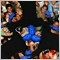# How do you use OnCalculate29

I refer to the sample given in the documentation. How are we meant to use OnCalculate?

1. With the old "start" event, we have a for loop to calculate the indicator per bar, how do we do that here with OnCalculate?
2. why are these arguments have an ampersand, e.g. &open[]? What is the difference with the normal open[]?
3. why do they need to be declared here as constants? Aren't open[], close[], etc are already available normally outside having to do OnCalculate?
4. why return(rates_total)?
5. Does anyone have a sample working indicator with OnCalculate?

THANK YOU

 int OnCalculate(const int rates_total,                const int prev_calculated,                const datetime &time[],                const double &open[],                const double &high[],                const double &low[],                const double &close[],                const long &tick_volume[],                const long &volume[],                const int &spread[])  {//--- Get the number of bars available for the current symbol and chart period   int bars=Bars(Symbol(),0);   Print("Bars = ",bars,", rates_total = ",rates_total,",  prev_calculated = ",prev_calculated);   Print("time = ",time," time[rates_total-1] = ",time[rates_total-1]);//--- return value of prev_calculated for next call   return(rates_total);  }840

When posting small portions of code, please make use of the SRC button.  If it is a large amount of code, please use "Attach file" and add the entire MQL file.53

JD4:
When posting small portions of code, please make use of the SRC button.  If it is a large amount of code, please use "Attach file" and add the entire MQL file.

Hello Angus,